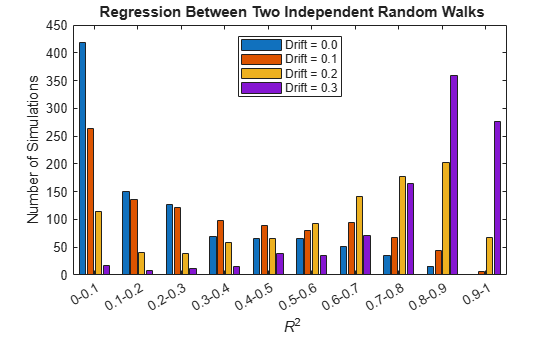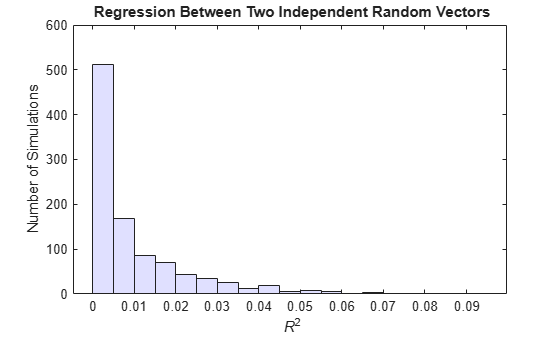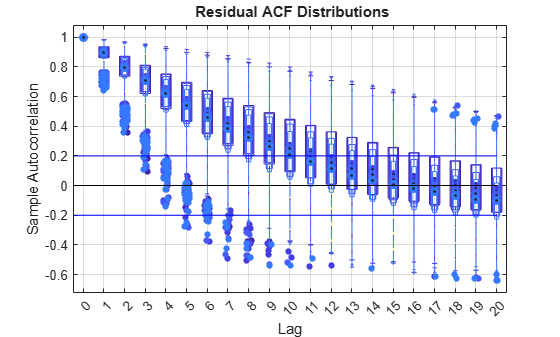Documentation

## Time Series Regression IV: Spurious Regression

This example considers trending variables, spurious regression, and methods of accommodation in multiple linear regression models. It is the fourth in a series of examples on time series regression, following the presentation in previous examples.

### Introduction

Predictors that trend over time are sometimes viewed with suspicion in multiple linear regression (MLR) models. Individually, however, they need not affect ordinary least squares (OLS) estimation. In particular, there is no need to linearize and detrend each predictor. If response values are well-described by a linear combination of the predictors, an MLR model is still applicable, and classical linear model (CLM) assumptions are not violated.

If, however, a trending predictor is paired with a trending response, there is the possibility of spurious regression, where $t$-statistics and overall measures of fit become misleadingly "significant." That is, the statistical significance of relationships in the model do not accurately reflect the causal significance of relationships in the data-generating process (DGP).

To investigate, we begin by loading relevant data from the previous example on "Influential Observations," and continue the analysis of the credit default model presented there:

load Data_TSReg3

### Confounding

One way that mutual trends arise in a predictor and a response is when both variables are correlated with a causally prior confounding variable outside of the model. The omitted variable (OV) becomes a part of the innovations process, and the model becomes implicitly restricted, expressing a false relationship that would not exist if the OV were included in the specification. Correlation between the OV and model predictors violates the CLM assumption of strict exogeneity.

When a model fails to account for a confounding variable, the result is omitted variable bias, where coefficients of specified predictors over-account for the variation in the response, shifting estimated values away from those in the DGP. Estimates are also inconsistent, since the source of the bias does not disappear with increasing sample size. Violations of strict exogeneity help model predictors track correlated changes in the innovations, producing overoptimistically small confidence intervals on the coefficients and a false sense of goodness of fit.

To avoid underspecification, it is tempting to pad out an explanatory model with control variables representing a multitude of economic factors with only tenuous connections to the response. By this method, the likelihood of OV bias would seem to be reduced. However, if irrelevant predictors are included in the model, the variance of coefficient estimates increases, and so does the chance of false inferences about predictor significance. Even if relevant predictors are included, if they do not account for all of the OVs, then the bias and inefficiency of coefficient estimates may increase or decrease, depending, among other things, on correlations between included and excluded variables . This last point is usually lost in textbook treatments of OV bias, which typically compare an underspecified model to a practically unachievable fully-specified model.

Without experimental designs for acquiring data, and the ability to use random sampling to minimize the effects of misspecification, econometricians must be very careful about choosing model predictors. The certainty of underspecification and the uncertain logic of control variables makes the role of relevant theory especially important in model specification. Examples in this series on "Predictor Selection" and "Residual Diagnostics" describe the process in terms of cycles of diagnostics and respecification. The goal is to converge to an acceptable set of coefficient estimates, paired with a series of residuals from which all relevant specification information has been distilled.

In the case of the credit default model introduced in the example on "Linear Models," confounding variables are certainly possible. The candidate predictors are somewhat ad hoc, rather than the result of any fundamental accounting of the causes of credit default. Moreover, the predictors are proxies, dependent on other series outside of the model. Without further analysis of potentially relevant economic factors, evidence of confounding must be found in an analysis of model residuals.

### Detrending

Detrending is a common preprocessing step in econometrics, with different possible goals. Often, economic series are detrended in an attempt to isolate a stationary component amenable to ARMA analysis or spectral techniques. Just as often, series are detrended so that they can be compared on a common scale, as with per capita normalizations to remove the effect of population growth. In regression settings, detrending may be used to minimize spurious correlations.

A plot of the credit default data (see the example on "Linear Models") shows that the predictor BBB and the response IGD are both trending. It might be hoped that trends could be removed by deleting a few atypical observations from the data. For example, the trend in the response seems mostly due to the single influential observation in 2001:

figure hold on plot(dates,y0,'k','LineWidth',2); plot(dates,y0-detrend(y0),'m.-') plot(datesd1,yd1-detrend(yd1),'g*-') hold off legend(respName0,'Trend','Trend with 2001 deleted','Location','NW') xlabel('Year') ylabel('Response Level') title('{\bf Response}') axis tight grid onDeleting the point reduces the trend, but does not eliminate it.

Alternatively, variable transformations are used to remove trends. This may improve the statistical properties of a regression model, but it complicates analysis and interpretation. Any transformation alters the economic meaning of a variable, favoring the predictive power of a model over explanatory simplicity.

The manner of trend-removal depends on the type of trend. One type of trend is produced by a trend-stationary (TS) process, which is the sum of a deterministic trend and a stationary process. TS variables, once identified, are often linearized with a power or log transformation, then detrended by regressing on time. The detrend function, used above, removes the least-squares line from the data. This transformation often has the side effect of regularizing influential observations.

### Stochastic Trends

Not all trends are TS, however. Difference stationary (DS) processes, also known as integrated or unit root processes, may exhibit stochastic trends, without a TS decomposition. When a DS predictor is paired with a DS response, problems of spurious regression appear . This is true even if the series are generated independently from one another, without any confounding. The problem is complicated by the fact that not all DS series are trending.

Consider the following regressions between DS random walks with various degrees of drift. The coefficient of determination (${R}^{2}$) is computed in repeated realizations, and the distribution displayed. For comparison, the distribution for regressions between random vectors (without an autoregressive dependence) is also displayed:

T = 100; numSims = 1000; drifts = [0 0.1 0.2 0.3]; numModels = length(drifts); Steps = randn(T,2,numSims); % Regression between two random walks: ResRW = zeros(numSims,T,numModels); RSqRW = zeros(numSims,numModels); for d = 1:numModels for s = 1:numSims Y = zeros(T,2); for t = 2:T Y(t,:) = drifts(d) + Y(t-1,:) + Steps(t,:,s); end % The compact regression formulation: % % MRW = fitlm(Y(:,1),Y(:,2)); % ResRW(s,:,d) = MRW.Residuals.Raw'; % RSqRW(s,d) = MRW.Rsquared.Ordinary; % % is replaced by the following for % efficiency in repeated simulation: X = [ones(size(Y(:,1))),Y(:,1)]; y = Y(:,2); Coeff = X\y; yHat = X*Coeff; res = y-yHat; yBar = mean(y); regRes = yHat-yBar; SSR = regRes'*regRes; SSE = res'*res; SST = SSR+SSE; RSq = 1-SSE/SST; ResRW(s,:,d) = res'; RSqRW(s,d) = RSq; end end % Plot R-squared distributions: figure [v(1,:),edges] = histcounts(RSqRW(:,1)); for i=2:size(RSqRW,2) v(i,:) = histcounts(RSqRW(:,i),edges); end numBins = size(v,2); ax = axes; ticklocs = edges(1:end-1)+diff(edges)/2; names = cell(1,numBins); for i = 1:numBins names{i} = sprintf('%0.5g-%0.5g',edges(i),edges(i+1)); end bar(ax,ticklocs,v.'); set(ax,'XTick',ticklocs,'XTickLabel',names,'XTickLabelRotation',30); fig = gcf; CMap = fig.Colormap; Colors = CMap(linspace(1,64,numModels),:); legend(strcat({'Drift = '},num2str(drifts','%-2.1f')),'Location','North') xlabel('{\it R}^2') ylabel('Number of Simulations') title('{\bf Regression Between Two Independent Random Walks}')clear RsqRW % Regression between two random vectors: RSqR = zeros(numSims,1); for s = 1:numSims % The compact regression formulation: % % MR = fitlm(Steps(:,1,s),Steps(:,2,s)); % RSqR(s) = MR.Rsquared.Ordinary; % % is replaced by the following for % efficiency in repeated simulation: X = [ones(size(Steps(:,1,s))),Steps(:,1,s)]; y = Steps(:,2,s); Coeff = X\y; yHat = X*Coeff; res = y-yHat; yBar = mean(y); regRes = yHat-yBar; SSR = regRes'*regRes; SSE = res'*res; SST = SSR+SSE; RSq = 1-SSE/SST; RSqR(s) = RSq; end % Plot R-squared distribution: figure histogram(RSqR) ax = gca; ax.Children.FaceColor = [.8 .8 1]; xlabel('{\it R}^2') ylabel('Number of Simulations') title('{\bf Regression Between Two Independent Random Vectors}')clear RSqR

The ${R}^{2}$ for the random-walk regressions becomes more significant as the drift coefficient increases. Even with zero drift, random-walk regressions are more significant than regressions between random vectors, where ${R}^{2}$ values fall almost exclusively below 0.1.

Spurious regressions are often accompanied by signs of autocorrelation in the residuals, which can serve as a diagnostic clue. The following shows the distribution of autocorrelation functions (ACF) for the residual series in each of the random-walk regressions above:

numLags = 20; ACFResRW = zeros(numSims,numLags+1,numModels); for s = 1:numSims for d = 1:numModels ACFResRW(s,:,d) = autocorr(ResRW(s,:,d)); end end clear ResRW % Plot ACF distributions: figure boxplot(ACFResRW(:,:,1),'PlotStyle','compact','BoxStyle','outline','LabelOrientation','horizontal','Color',Colors(1,:)) ax = gca; ax.XTickLabel = {''}; hold on boxplot(ACFResRW(:,:,2),'PlotStyle','compact','BoxStyle','outline','LabelOrientation','horizontal','Widths',0.4,'Color',Colors(2,:)) ax.XTickLabel = {''}; boxplot(ACFResRW(:,:,3),'PlotStyle','compact','BoxStyle','outline','LabelOrientation','horizontal','Widths',0.3,'Color',Colors(3,:)) ax.XTickLabel = {''}; boxplot(ACFResRW(:,:,4),'PlotStyle','compact','BoxStyle','outline','LabelOrientation','horizontal','Widths',0.2,'Color',Colors(4,:),'Labels',0:20) line([0,21],[0,0],'Color','k') line([0,21],[2/sqrt(T),2/sqrt(T)],'Color','b') line([0,21],[-2/sqrt(T),-2/sqrt(T)],'Color','b') hold off xlabel('Lag') ylabel('Sample Autocorrelation') title('{\bf Residual ACF Distributions}') grid onclear ACFResRW

Colors correspond to drift values in the bar plot above. The plot shows extended, significant residual autocorrelation for the majority of simulations. Diagnostics related to residual autocorrelation are discussed further in the example on "Residual Diagnostics."

### Differencing

The simulations above lead to the conclusion that, trending or not, all regression variables should be tested for integration. It is then usually advised that DS variables be detrended by differencing, rather than regressing on time, to achieve a stationary mean.

The distinction between TS and DS series has been widely studied (for example, in ), particularly the effects of underdifferencing (treating DS series as TS) and overdifferencing (treating TS series as DS). If one trend type is treated as the other, with inappropriate preprocessing to achieve stationarity, regression results become unreliable, and the resulting models generally have poor forecasting ability, regardless of the in-sample fit.

Econometrics Toolbox™ has several tests for the presence or absence of integration: adftest, pptest, kpsstest, and lmctest. For example, the augmented Dickey-Fuller test, adftest, looks for statistical evidence against a null of integration. With default settings, tests on both IGD and BBB fail to reject the null in favor of a trend-stationary alternative:

IGD = y0; BBB = X0(:,2); [h1IGD,pValue1IGD] = adftest(IGD,'model','TS')
h1IGD = logical 0 
pValue1IGD = 0.1401 
[h1BBB,pValue1BBB] = adftest(BBB,'model','TS')
h1BBB = logical 0 
pValue1BBB = 0.6976 

Other tests, like the KPSS test, kpsstest, look for statistical evidence against a null of trend-stationarity. The results are mixed:

s = warning('off'); % Turn off large/small statistics warnings [h0IGD,pValue0IGD] = kpsstest(IGD,'trend',true)
h0IGD = logical 0 
pValue0IGD = 0.1000 
[h0BBB,pValue0BBB] = kpsstest(BBB,'trend',true)
h0BBB = logical 1 
pValue0BBB = 0.0100 

The p-values of 0.1 and 0.01 are, respectively, the largest and smallest in the table of critical values used by the right-tailed kpsstest. They are reported when the test statistics are, respectively, very small or very large. Thus the evidence against trend-stationarity is especially weak in the first test, and especially strong in the second test. The IGD results are ambiguous, failing to reject trend-stationarity even after the Dickey-Fuller test failed to reject integration. The results for BBB are more consistent, suggesting the predictor is integrated.

What is needed for preprocessing is a systematic application of these tests to all of the variables in a regression, and their differences. The utility function i10test automates the required series of tests. The following performs paired ADF/KPSS tests on all of the model variables and their first differences:

I.names = {'model'}; I.vals = {'TS'}; S.names = {'trend'}; S.vals = {true}; i10test(DataTable,'numDiffs',1,... 'itest','adf','iparams',I,... 'stest','kpss','sparams',S);
Test Results I(1) I(0) ====================== AGE 1 0 0.0069 0.1000 D1AGE 1 0 0.0010 0.1000 ---------------------- BBB 0 1 0.6976 0.0100 D1BBB 1 0 0.0249 0.1000 ---------------------- CPF 0 0 0.2474 0.1000 D1CPF 1 0 0.0064 0.1000 ---------------------- SPR 0 1 0.2563 0.0238 D1SPR 1 0 0.0032 0.1000 ---------------------- IGD 0 0 0.1401 0.1000 D1IGD 1 0 0.0028 0.1000 ---------------------- 
warning(s) % Restore warning state

Columns show test results and p-values against nulls of integration, $I\left(1\right)$, and stationarity, $I\left(0\right)$. At the given parameter settings, the tests suggest that AGE is stationary (integrated of order 0), and BBB and SPR are integrated but brought to stationarity by a single difference (integrated of order 1). The results are ambiguous for CPF and IGD, but both appear to be stationary after a single difference.

For comparison with the original regression in the example on "Linear Models," we replace BBB, SPR, CPF, and IGD with their first differences, D1BBB, D1SPR, D1CPF, and D1IGD. We leave AGE undifferenced:

D1X0 = diff(X0); D1X0(:,1) = X0(2:end,1); % Use undifferenced AGE D1y0 = diff(y0); predNamesD1 = {'AGE','D1BBB','D1CPF','D1SPR'}; respNameD1 = {'D1IGD'};

Original regression with undifferenced data:

M0
M0 = Linear regression model: IGD ~ 1 + AGE + BBB + CPF + SPR Estimated Coefficients: Estimate SE tStat pValue _________ _________ _______ _________ (Intercept) -0.22741 0.098565 -2.3072 0.034747 AGE 0.016781 0.0091845 1.8271 0.086402 BBB 0.0042728 0.0026757 1.5969 0.12985 CPF -0.014888 0.0038077 -3.91 0.0012473 SPR 0.045488 0.033996 1.338 0.1996 Number of observations: 21, Error degrees of freedom: 16 Root Mean Squared Error: 0.0763 R-squared: 0.621, Adjusted R-Squared: 0.526 F-statistic vs. constant model: 6.56, p-value = 0.00253 

Regression with differenced data:

MD1 = fitlm(D1X0,D1y0,'VarNames',[predNamesD1,respNameD1])
MD1 = Linear regression model: D1IGD ~ 1 + AGE + D1BBB + D1CPF + D1SPR Estimated Coefficients: Estimate SE tStat pValue _________ _________ ________ _________ (Intercept) -0.089492 0.10843 -0.82535 0.4221 AGE 0.015193 0.012574 1.2083 0.24564 D1BBB -0.023538 0.020066 -1.173 0.25909 D1CPF -0.015707 0.0046294 -3.393 0.0040152 D1SPR -0.03663 0.04017 -0.91187 0.37626 Number of observations: 20, Error degrees of freedom: 15 Root Mean Squared Error: 0.106 R-squared: 0.49, Adjusted R-Squared: 0.354 F-statistic vs. constant model: 3.61, p-value = 0.0298 

The differenced data increases the standard errors on all coefficient estimates, as well as the overall RMSE. This may be the price of correcting a spurious regression. The sign and the size of the coefficient estimate for the undifferenced predictor, AGE, shows little change. Even after differencing, CPF has pronounced significance among the predictors. Accepting the revised model depends on practical considerations like explanatory simplicity and forecast performance, evaluated in the example on "Forecasting."

### Summary

Because of the possibility of spurious regression, it is usually advised that variables in time series regressions be detrended, as necessary, to achieve stationarity before estimation. There are trade-offs, however, between working with variables that retain their original economic meaning and transformed variables that improve the statistical characteristics of OLS estimation. The trade-off may be difficult to evaluate, since the degree of "spuriousness" in the original regression cannot be measured directly. The methods discussed in this example will likely improve the forecasting abilities of resulting models, but may do so at the expense of explanatory simplicity.

### References

 Clarke, K. A. "The Phantom Menace: Omitted Variable Bias in Econometric Research." Conflict Management and Peace Science. Vol. 22, 2005, pp. 341-352.

 Granger, C., and P. Newbold. (1974). "Spurious Regressions in Econometrics". Journal of Econometrics. Vol. 2, 1974, pp. 111-120.

 Nelson, C. R., and C. I. Plosser. "Trends Versus Random Walks in Macroeconomic Time Series: Some Evidence and Implications." Journal of Monetary Economics. Vol. 10, 1982, pp. 139-162.